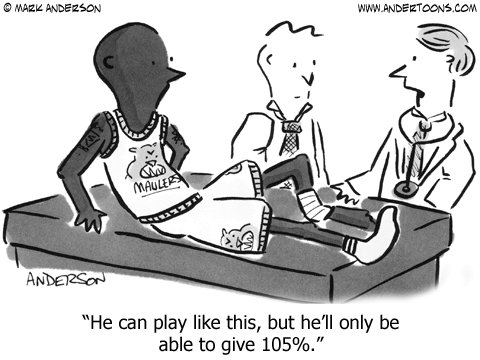## Spacey FictionAt home I put the book on the table, and walked away. Later, I walked by the book and decided to pick it up to read the first page just to get a feel for the story. That was the end of it for me. I never put it down again until bedtime.

The Martiani is the best book I have read in a long time. The premise was totally believable, the character was real, and totally funny. It takes quite a man to be funny when marooned on a planet eleven months from Earth. His attitude was one of survival, and he was expert at solving problems throughout. My background is in engineering and this story is loaded with heaps of technical jargon and science. All of it made sense and based on fact. This character put McGyver to shame.

Now that I love the story so much I will endure the movie to see if Damon did the story justice.

## PSA-170830-Mathematical LogicMathematics:

This comes from 2 math teachers with a combined total of 70 yrs. experience.
It has an indisputable mathematical logic.

This is a strictly …..  a mathematical viewpoint… and it goes like this:

What Makes 100%?
What does it mean to give MORE than 100%?
Ever wonder about those people who say they are giving more than 100%? We have all been to those meetings where someone wants you to give over 100%.
What makes up 100% in life?
If:
A B C D E F G H I J K L M N O P Q R S T U V W X Y Z
Is represented as:
1 2 3 4 5 6 7 8 9 10 11 12 13 14 15 16 17 18 19 20 21 22 23 24 25 26.
Then:
H-A-R-D-W-O-R-K
8+1+18+4+23+15+18+11 =  98%
And
K-N-O-W-L-E-D-G-E
11+14+15+23+12+5+4+7+5 =
96%
But ,
A-T-T-I-T-U-D-E
1+20+20+9+20+21+4+5 =  100%
And,
B-U-L-L-S-H-I-T
2+21+12+12+19+8+9+20 =  103%
AND, look how far ass kissing will take you.
A-S-S-K-I-S-S-I-N-G
1+19+19+11+9+19+19+9+14+7  = 118%
So, one can conclude with mathematical certainty, that while Hardwork and Knowledge will get you close, and Attitude will get you there.  It’s the Bullshit and good old fashion Ass Kissing that will put you over the top.
Now you know why Politicians are where they are!
I’ve never seen a better explanation than this formula. How true it is?